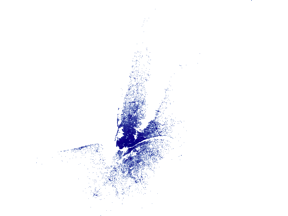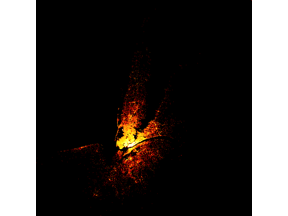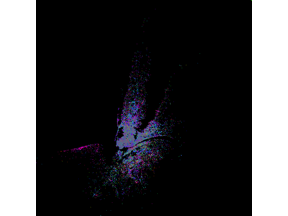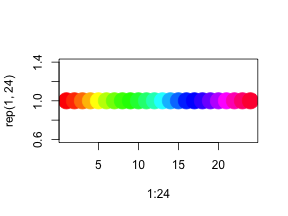``````library(rasterly)
library(data.table)
library(lubridate)
library(grid)
library(plotly)
``````

`rasterly` makes it easy to rapidly generate raster images for large datasets. Although the package is inspired by the Datashader library (http://datashader.org/getting_started/index.html) available for Python, `rasterly` does not attempt to reproduce all the features of Datashader.

Rather, `rasterly` offers comparable performance to Datashader when generating rasters from source data. `rasterly` attempts to provide a flexible, convenient interface which should feel familiar to users of ggplot2 and its aesthetics-based approach to customizing plots and figures.

## Data set

The dataset used in this vignette describes Uber trips taken in New York City from April 1st to September 30th of 2014.

``````# Load data
ridesRaw_1 <- "https://raw.githubusercontent.com/plotly/datasets/master/uber-rides-data1.csv" %>%
ridesRaw_2 <- "https://raw.githubusercontent.com/plotly/datasets/master/uber-rides-data2.csv" %>%
ridesRaw_3 <- "https://raw.githubusercontent.com/plotly/datasets/master/uber-rides-data3.csv"  %>%
ridesDf <- list(ridesRaw_1, ridesRaw_2, ridesRaw_3) %>%
data.table::rbindlist()

# Extract hour of trip taken
time <- lubridate::ymd_hms(ridesDf\$`Date/Time`)
ridesDf <-  ridesDf[, 'Date/Time':=NULL][, list(Lat,
Lon,
hour = lubridate::hour(time),
month = lubridate::month(time),
day = lubridate::day(time))]
``````
``````##        Lat      Lon hour month day
## 1: 40.7690 -73.9549    0     4   1
## 2: 40.7267 -74.0345    0     4   1
## 3: 40.7316 -73.9873    0     4   1
## 4: 40.7588 -73.9776    0     4   1
## 5: 40.7594 -73.9722    0     4   1
## 6: 40.7383 -74.0403    0     4   1
``````

This dataset has 4,533,327 observations, and includes the variables “latitude”, “longitude”, “hour”, “month” and “day”.

## Basic

If we were to use `graphics::plot()`, it would take several minutes to render the image. What if we “rasterized” the image instead?

``````start_time <- Sys.time()
p <- ridesDf %>%
rasterly(mapping = aes(x = Lat, y = Lon)) %>%
rasterize_points()
p
````````````end_time <- Sys.time()
end_time - start_time
``````
``````## Time difference of 0.1704991 secs
``````

## `rasterly` Structure

#### Subsetting

`rasterly()` generates a parent layer containing initial settings to generate the raster, which include `plot_height`, `plot_width` among others; child layers such as `rasterize_points()` can be piped in as well. Note that “p” above is a list of environments.

``````# A list of environments
str(p)
``````
``````## List of 2
##  \$ rasterly_env   :<environment: 0x7fc54ee5e190>
##  \$ rasterlyPoints1:<environment: 0x7fc54c1c1fb8>
##  - attr(*, "class")= chr [1:3] "rasterlyPoints" "rasterlyLayer" "rasterly"
``````

The elements in “p” can be easily extracted or replaced by `[` and `[<-`.

``````p["background"]
``````
``````## \$rasterly_env
##  "white"
##
## \$rasterlyPoints1
##  "white"
``````
``````# Replace the background in child layer `rasterly_points()`
p["background", level = 2] <- "black"
p["background"]
``````
``````## \$rasterly_env
##  "white"
##
## \$rasterlyPoints1
##  "black"
``````
``````# color_maps in both `rasterly()` and `rasterly_points()` are replaced
## fire_map is a vector of colors (as character strings) with length 256
## see `rasterly::fire_map`
p["color_map", level = 1:2] <- fire_map
p
``````• `level` helps to define which layer to replace; the default is `1` (the parent layer generated by `rasterly()`).
• Available states which can be extracted or replaced are listed here:
1. Aggregation: `data`, `mapping`, `plot_width`, `plot_height`, `range`, `x_range`, `y_range`, `xlim`, `ylim`, `aesthetics`, `reduction_func`, `glyph`, `max_size`, `group_by_data_table`, `drop_data`, `variable_check`
2. Display: `background`, `color_map`, `color_key`, `alpha`, `span`, `show_raster`, `layout`

#### Build rasterly by `rasterly_build()`

To retrieve display info, use `rasterly_build()`:

``````build <- rasterly_build(p)
str(build)
``````
``````## List of 10
##  \$ agg           :List of 1
##   ..\$ rasterlyPoints1:List of 1
##   .. ..\$ : 'rasterizeMatrix' num [1:600, 1:600] 0 0 0 0 0 0 0 0 0 0 ...
##  \$ image         : chr [1:600, 1:600] "black" "black" "black" "black" ...
##  \$ lims          :List of 1
##   ..\$ rasterlyPoints1:List of 2
##   .. ..\$ xlim: num [1:2] 39.7 42.1
##   .. ..\$ ylim: num [1:2] -74.9 -72.1
##  \$ x_range       : num [1:2] 39.7 42.1
##  \$ y_range       : num [1:2] -74.9 -72.1
##  \$ plot_height   : num 600
##  \$ plot_width    : num 600
##  \$ variable_names:List of 1
##   ..\$ rasterlyPoints1: Named chr [1:2] "Lat" "Lon"
##   .. ..- attr(*, "names")= chr [1:2] "x" "y"
##  \$ background    : chr "black"
##  \$ colors        :List of 1
##   ..\$ : chr [1:256] "#000000" "#060000" "#0d0000" "#120000" ...
##  - attr(*, "class")= chr [1:2] "rasterize" "rasterly"
``````

It contains:

• agg: aggregation matrices, a list of numerical matrices
• image: a raster matrix (has the same dimension with aggregation matrices)
• lims: a list of x limits and y limits for each layer
• x_range: the range of x over all layers
• y_range: the range of y over all layers
• plot_height: plot height, number of rows in aggregation matrix
• plot_width: plot width, number of columns in aggregation matrix
• variable_names: names of variables
• background: background color
• colors: color_map (color used to map in each pixel) or color_key (used for categorical variable. In general, 'color_key' would be called when “color” is set in `aes()`)

## Display

`rasterly` does not provide any functionality to display the raster image data it generates, but instead relies on other packages.

#### `plotly` graphics

• `add_rasterly_heatmap()`: Layers are added to Plotly objects via `add_trace(...)`; `rasterly` provides the `add_rasterly_heatmap()` function which also leverages `add_heatmap()` to generate single channel heatmap overlays for Plotly figures. Multi-channel heatmaps are not currently supported; this feature will be available in an upcoming release.

``````plotly::plot_ly(ridesDf, x = ~Lat, y = ~Lon) %>%
layout(
title = "Uber drives",
x = list(
title = "Lat"
),
y = list(
title = "Lon"
)
)
``````
``````## The default scaling is 'log'.
``````
``````## Warning: 'layout' objects don't have these attributes: 'x', 'y'
## Valid attributes include:
## 'font', 'title', 'autosize', 'width', 'height', 'margin', 'paper_bgcolor', 'plot_bgcolor', 'separators', 'hidesources', 'showlegend', 'colorway', 'datarevision', 'uirevision', 'editrevision', 'selectionrevision', 'template', 'modebar', 'meta', 'transition', '_deprecated', 'clickmode', 'dragmode', 'hovermode', 'hoverdistance', 'spikedistance', 'hoverlabel', 'selectdirection', 'grid', 'calendar', 'xaxis', 'yaxis', 'ternary', 'scene', 'geo', 'mapbox', 'polar', 'radialaxis', 'angularaxis', 'direction', 'orientation', 'editType', 'legend', 'annotations', 'shapes', 'images', 'updatemenus', 'sliders', 'colorscale', 'coloraxis', 'metasrc', 'barmode', 'bargap', 'mapType'
``````• `plotly.rasterly()`: `plotly.rasterly` takes a `rasterly` object and returns a “plotly” object:

``````# plotly
ply <- p %>%
plotly.rasterly(sizing = "contain")
ply
``````

## API

`rasterly` application programming interface

``````r <- rasterly(data = ridesDf,
mapping = aes(x = Lat, y = Lon))
``````

#### Mapping system

• Set `color`
``````r %>%
rasterize_points(
mapping = aes(color = hour),
color_key = hourColors_map,
background = "black"
) -> g
g
``````Different colors represent different hours:

``````# rasterly doesn't currently support legends, though this feature is forthcoming
plot(1:24, y = rep(1,24), col = hourColors_map, pch = 19, cex = 3)
``````The number of aggregation matrices is equivalent to the number of categories:

``````build_g <- rasterly_build(g)
# the object has only one layer, so we index into the first element
length(build_g\$agg[])
``````
``````##  24
``````
``````# 24
``````

The colors attribute in “image” within `build_g` is generated via weighted arithmetic means (default) computed from the aggregation matrices. We can choose the “cover” layout to display multiple aggregation matrices:

``````r %>%
rasterize_points(
mapping = aes(color = hour),
color_key = hourColors_map,
background = "black",
layout = "cover"
)
``````

The resulting raster will be overlaid onto the plotting surface.

• Set `on`

`reduction_func` is implemented `on` which variable

``````r %>%
rasterize_points(
reduction_func = "mean", # take the "mean" reduction function
mapping = aes(on = -Lat)
)
``````
• Set `size`

To control the number of pixels allocated to an observation, we can set the `size` aesthetic; when specified, the `max_size` argument provides the upper bound of the number of pixels a single observation is allocated:

``````r %>%
rasterize_points(
mapping = aes(size = month),
max_size = 4
)
``````

Currently, only `x`, `y`, `color`, `on` and `size` can be set using `aes()`.

#### Reduction function

A reduction operator function is used when aggregating data points within each bin. One option is to reduce using the mean of the points.

• `mean` reduction function:
``````r %>%
rasterize_points(
reduction_func = "mean", # process the data points using the mean reduction function
background = "black",    # change background to "black" from right to left (from dark to light)
color_map = fire_map # provide a custom color_map
)
``````

The `mean` reduction function averages the y column (default setting) for every observation. It's also possible to average over other features using the `on` aesthetic; consult the list of available reduction functions below for additional details.

`on`

• `any` reduction function:
``````r %>%
rasterize_points(
reduction_func = "any",
color_map = c("white", "black")
)
``````

Currently supported reduction functions:

• `sum`: If `on` is not provided within `aes()`, the default is to take the sum within each bin. When `on` is specified, the function reduces by taking the sum of all elements within the variable named in `on`.

• `any`: When `on` is provided within `aes()`, the `any` reduction function specifies whether any elements in `on` should be mapped to each bin.

• `mean`: If `on` is not provided in mapping `aes()`, `on` would be set as variable “y” by default. When `on` is given, the `mean` reduction function takes the mean of all elements within the variable specified by `on`.

The following functions require that `on` is first provided via `aes()`:

• `m2`: The `m2` function computes the sum of square differences from the mean of all elements in the variable specified by `on`.

• `var`: The `var` function computes the variance over all elements in the vector specified by `on`.

• `sd`: The `sd` function computes the standard deviation over all elements in the vector specified by `on`.

• `first`: The `first` function returns the first element in the vector specified by `on`.

• `last`: The `last` function returns the last element in the vector specified by `on`.

• `min`: The `min` function returns the minimum value in the vector specified by `on`.

• `max`: The `min` function returns the maximum value in the vector specified by `on`.

`rasterly` is in active development; please report issues and request features via https://github.com/plotly/rasterly/issues.

Future work: provide support for `ggplot2` via `geom_rasterly()`, and `loon` via `l_rasterly()`.